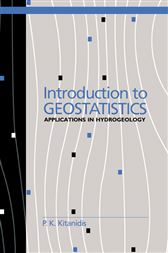# Introduction to Geostatistics

## Applications in HydrogeologyAU\$62.70
Engineers and applied geophysicists routinely encounter interpolation and estimation problems when analysing data from field observations. Introduction to Geostatistics presents practical techniques for the estimation of spatial functions from sparse data. The author's unique approach is a synthesis of classic and geostatistical methods with a focus on the most practical linear minimum-variance estimation methods, and includes suggestions on how to test and extend the applicability of such methods. The author includes many useful methods (often not covered in other geostatistics books) such as estimating variogram parameters, evaluating the need for a variable mean, parameter estimation and model testing in complex cases (e.g. anisotropy, variable mean, and multiple variables), and using information from deterministic mathematical models. Well illustrated with exercises and worked examples taken from hydrogeology, Introduction to Geostatistics assumes no background in statistics and is suitable for graduate-level courses in earth sciences, hydrology, and environmental engineering, and also for self-study.

• Cambridge University Press; May 1997
• ISBN: 9780511823480